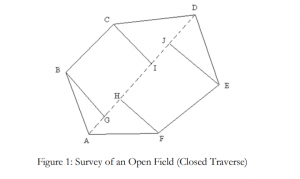# SURVEY OF AN AREA BY CHAIN SURVEY(CLOSED TRAVERSE)

## SURVEY OF AN AREA BY CHAIN SURVEY(CLOSED TRAVERSE)

In this post, I am going to discuss about to survey an open field by in order to calculate the area of the open field.

### Equipment used to find the area using chain surveying

Chain, Tape, Ranging Rods, Arrows, Cross Staff.

## Procedure for surveying the given open field (Closed Traverse)

Note: This procedure is a general procedure only. This procedure varies with the experiment given to students. Therefore students are required to write the procedure according to the experiment given to them.
Example 1:
1. ABCDEF is the required closed traverse open field to be surveyed for calculating the area as shown in Fig 1.
2. From the station A the length of all the opposite corners such as AC, AD and AE are measured with a chain and the longest distance is considered for laying off the main chain line. In this case AD is the longest and a chain line running from A to D is laid.
3. Offsets to corner points B, C, E and F are now laid from the chain line AD either by tape or cross-staff and their foot of offsets are G, I, J, H respectively.
4. All the offset lengths GB, HF, IC and JE are measured either by chain or tape depending on the length of offsets.
5. The distances between all the points AG, GH, HI, IJ and JD are also measured along the chain line.
6. Area Calculations: (Note: Areas of all triangles and trapeziums are calculated and added together to calculate the total area of open field (Closed Traverse) as described in class).Example 2
1. Let ABCDE be the given field whose area is to be measured, fix the pegs at A, B, C, D & E.
2. Divide area into three triangles ADE, ABD and BCD by joining AD and BD.
3. Measure the lengths AB, BC, CD, DE, EA, AD and BD.
4. Calculate the area of the triangles.
5. The sum of the areas of the three triangles is the area of the given field.

### The formula for calculating the area of the plot by chain surveying

Area of the triangle Δ = √ s (s-a) (s-b) (s-c) ( Root of )
Where S = (a + b+ c) / 2
a, b, c, are the sides of the triangle.

Sketch of the plotThis is the method to survey an area by chain surveying process.

Open